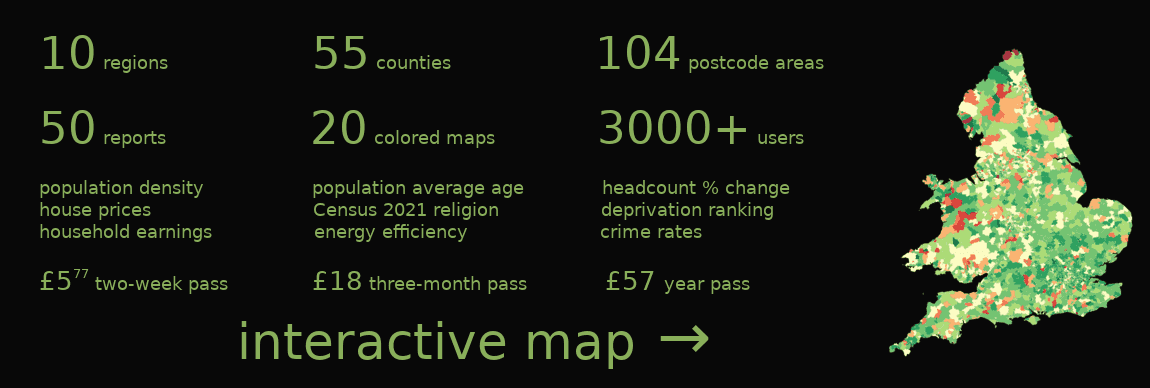# York population changes

In 2020, the total population of York postcode area was 591k and it increased by 60.1k people since 2002. That's 11.3% population growth . Population average age was 43.6 and it increased by 2.6 years since 2002. The postcode area was ageing faster than England and Wales in which the age grew by 1.7 years in the same period. The population was growing slower than population in England and Wales which grew by 13.5%.

# +60.1k

population growth since 2002

# +11.3%

population growth since 2002

# +2.6 yrs

average age change
since 2002

Compares speed of population growth to other postcode areas. Areas with the highest population growth are on the left. Shows relative population change between 2002 and 2020.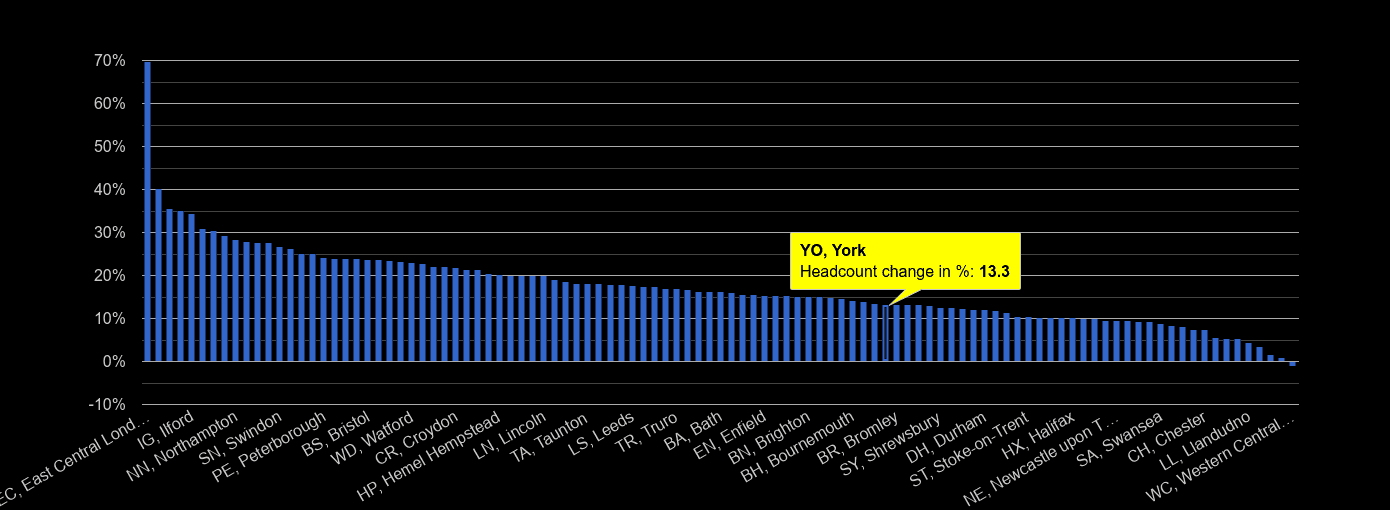Compares the latest population estimates with population estimates in 2002 and shows percentage difference.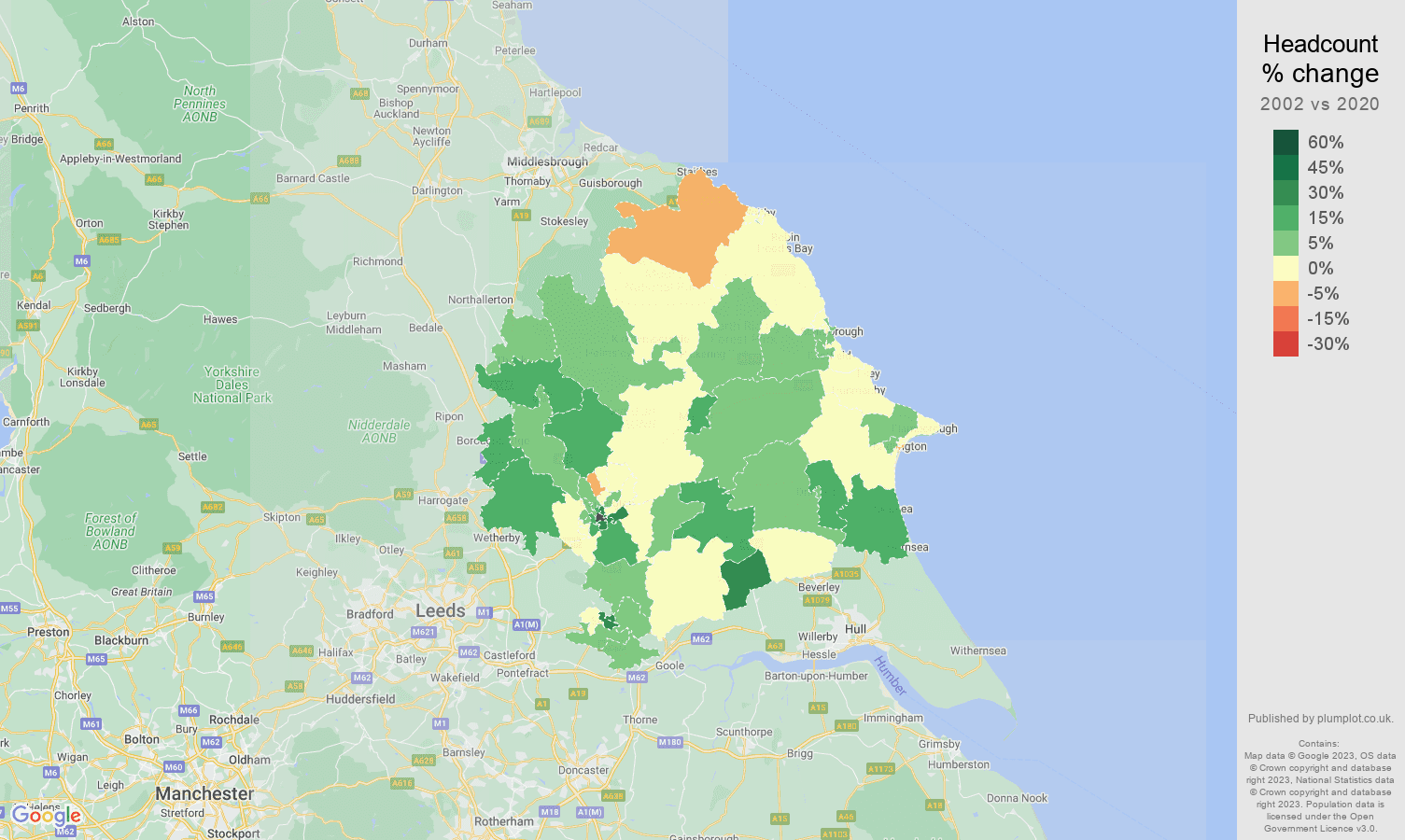## York population growth rate

Shows year over year population change.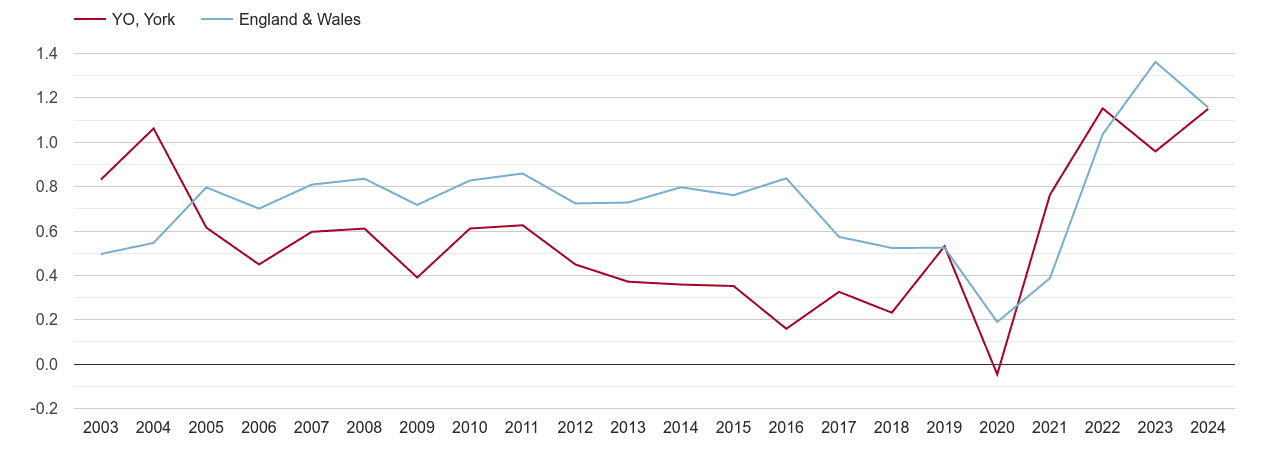## York population growth

Shows total population by year.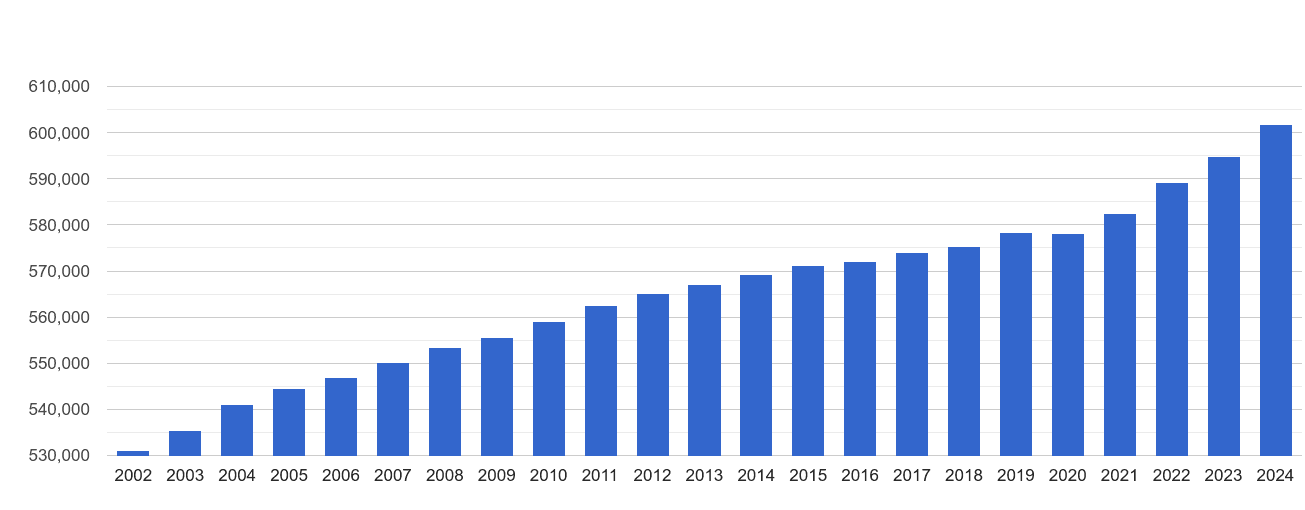## York average age change map

Shows population's average age change since 2002.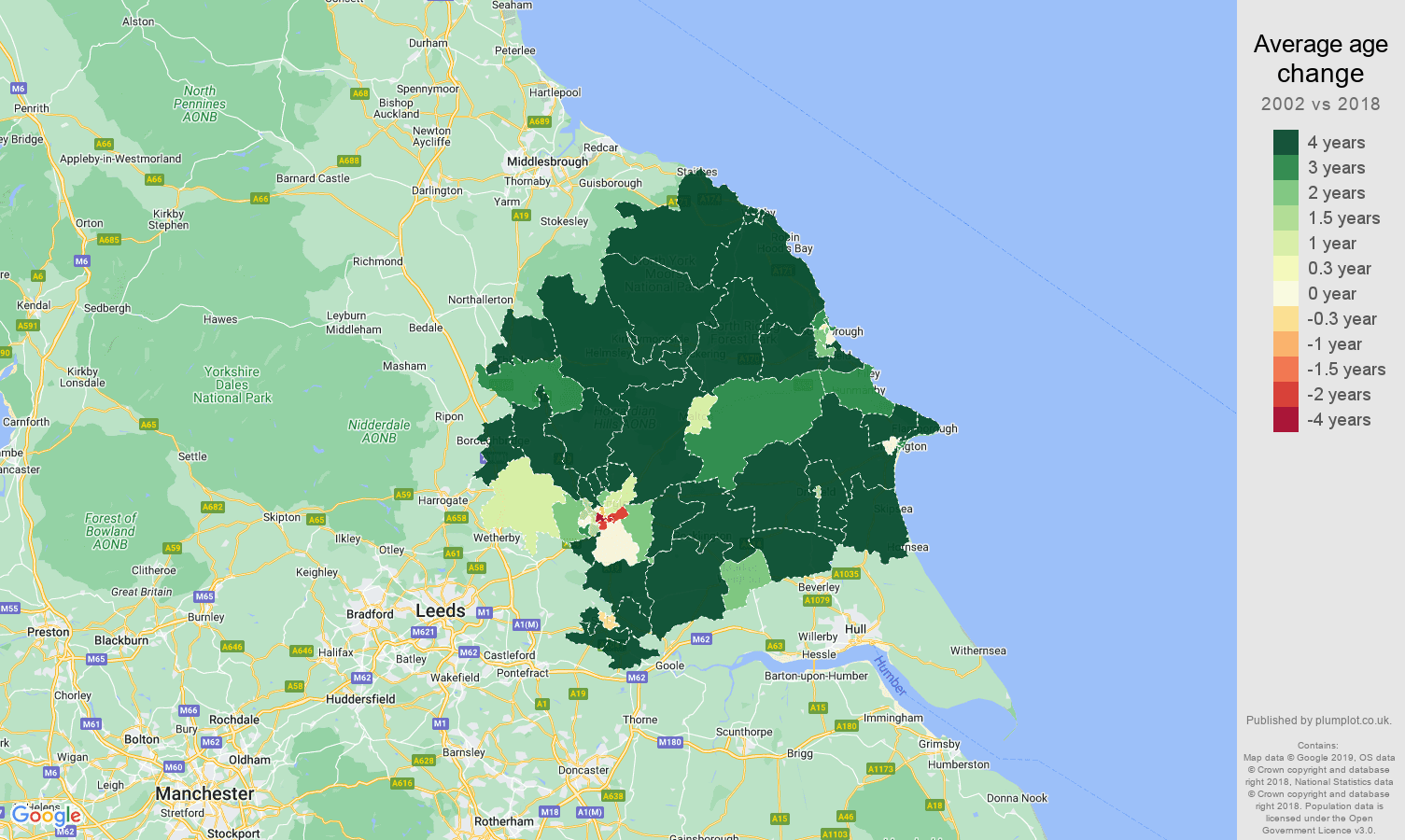## York population average age change rank

Compares the speed of average age change in the postcode areas. Areas with the fastest ageing population are on the left, the areas with their population becoming younger are on the right. Compares change between 2002 and 2020.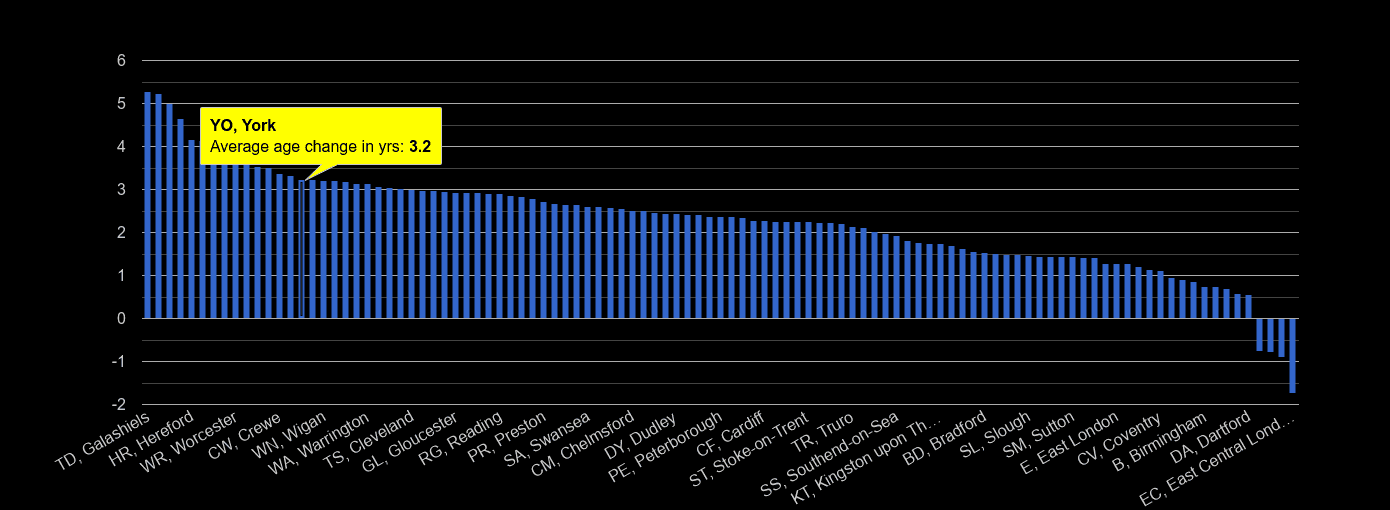## York population average age

Annual statistics of population's average age.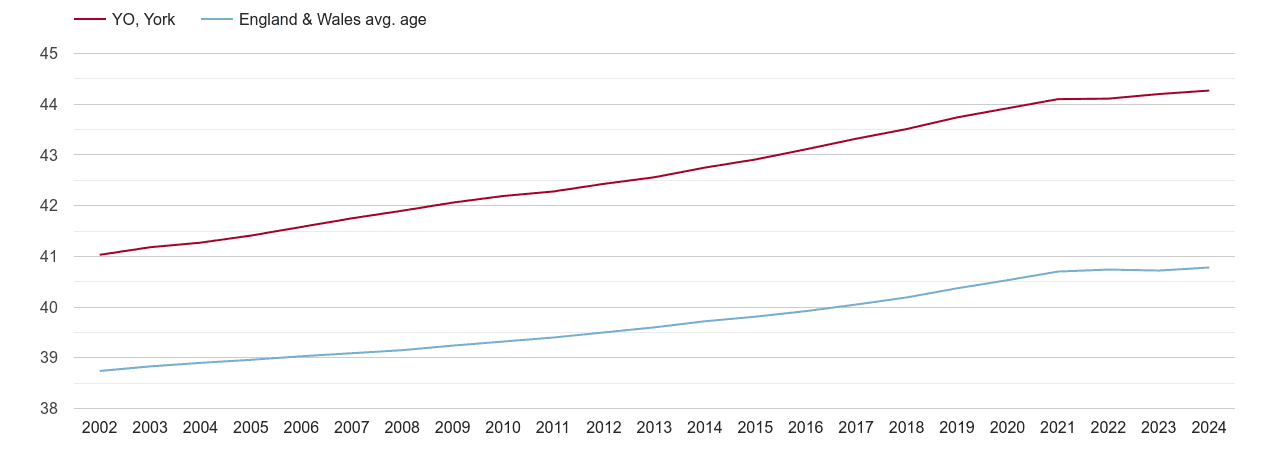## York male and female average age

Annual statistics of population's average age by sex.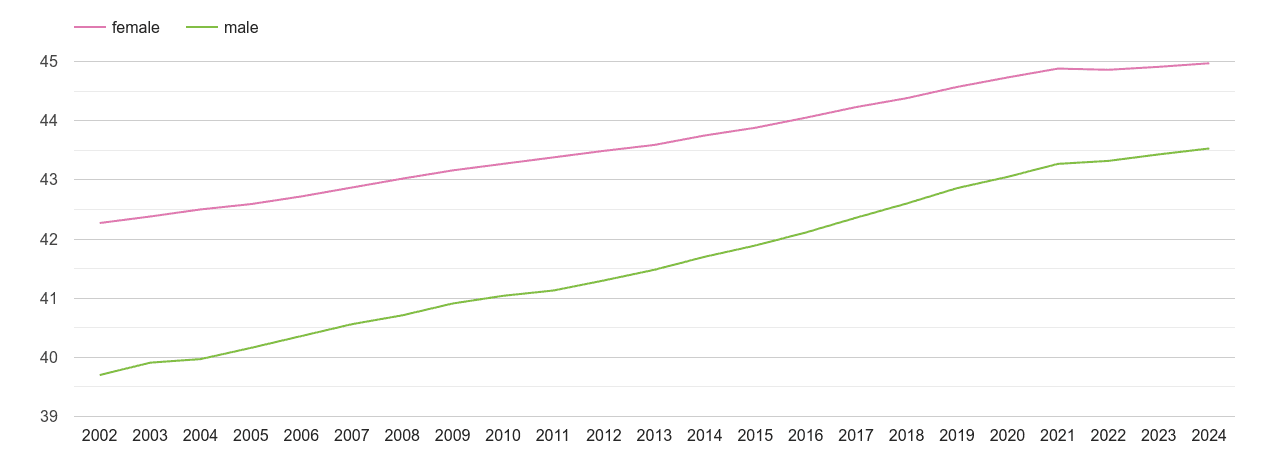## York population changes compared to other areas

#### Comparison to other postcode areas. Metrics show a percentage change in the headcount between 2002 and 2020 on y-axis and change of the population's average age on x-axis. The size of the circle is directly proportional to the total population in 2020.

Population average age change

## Plumplot area insights - interactive charts and maps